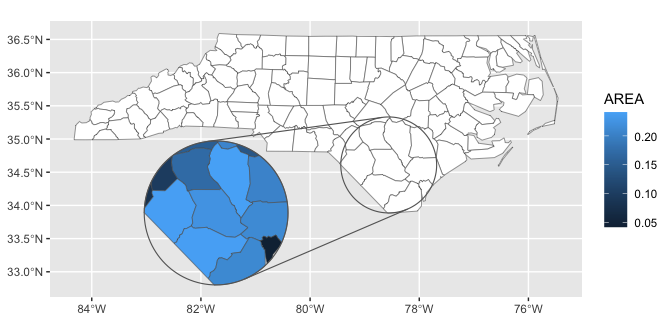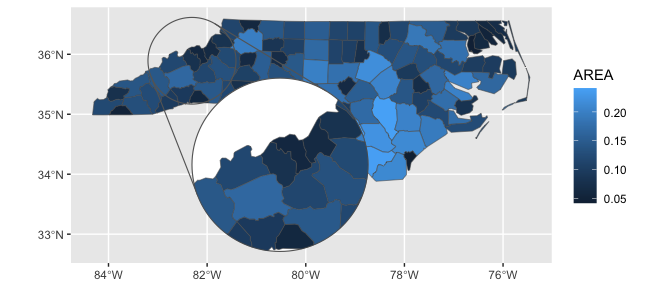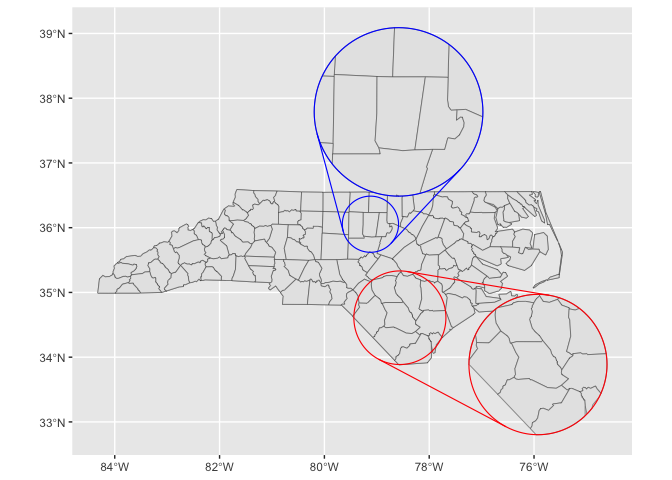# Getting started with ‘ggmapinset’

library(ggmapinset)
#> Linking to GEOS 3.11.2, GDAL 3.6.4, PROJ 9.2.0; sf_use_s2() is TRUE
library(ggplot2)

nc <- sf::st_read(system.file("shape/nc.shp", package = "sf"), quiet = TRUE)

## Different aesthetics for inset layer

By default, geom_sf_inset() creates two copies of the map layer: one for the base map and the other for the inset map. The inset is transformed and clipped, but uses the same underlying aesthetics mapping and parameters.

If you want to have different aesthetics for the two layers, you’ll need to turn off this copying with map_base = "none". With this parameter set and an inset parameter provided, only the inset layer will be drawn. To draw only the base layer, you can use map_inset = "none", inset = NULL, or simply use the normal geom_sf().

ggplot(nc) +
# this is equivalent to the following line:
# geom_sf_inset(fill = "white", map_inset = "none") +
geom_sf(fill = "white") +
geom_sf_inset(aes(fill = AREA), map_base = "none") +
geom_inset_frame() +
coord_sf_inset(inset = configure_inset(
centre = sf::st_centroid(sf::st_geometry(nc)[nc$NAME == "Bladen"]), scale = 1.5, translation = c(-180, -50), radius = 50, units = "mi"))## Inset frame backgrounds By default, the inset frame is transparent, although often it makes sense to add a solid background so that the inset is distinguishable from any overlapping part of the base map. The aesthetics of the two parts of the frame and the burst lines connecting them can be controlled separately. Note that when the background is filled, we need to specify the base and inset maps in separate layers so that the frame can slip in between them. ggplot(nc) + geom_sf(aes(fill = AREA)) + geom_inset_frame(target.aes = list(fill = "white")) + geom_sf_inset(aes(fill = AREA), map_base = "none") + coord_sf_inset(inset = configure_inset( centre = st_centroid(st_geometry(nc)[nc$NAME == "Yancey"]), scale = 2,
translation = c(100, -120), radius = 50, units = "mi"))## Multiple insets

For multiple insets, the appropriate inset configuration just needs to be passed to each layer separately. It’s probably clearer to avoid providing an inset to the coordinate system in this case.

Since the inset-aware layers will duplicate themselves for the base and inset maps, you will probably want to disable that behaviour with map_base = "none" to avoid having multiple identical copies of the base map.

inset1 <- configure_inset(
centre = sf::st_centroid(sf::st_geometry(nc)[nc$NAME == "Bladen"]), scale = 1.5, translation = c(150, -50), radius = 50, units = "mi") inset2 <- configure_inset( centre = sf::st_centroid(sf::st_geometry(nc)[nc$NAME == "Orange"]), scale = 3,
translation = c(30, 120), radius = 30, units = "mi")

ggplot(nc) +
# base map
geom_sf_inset() +
# inset 1
geom_sf_inset(map_base = "none", inset = inset1) +
geom_inset_frame(inset = inset1, colour = "red") +
# inset 2
geom_sf_inset(map_base = "none", inset = inset2) +
geom_inset_frame(inset = inset2, colour = "blue")